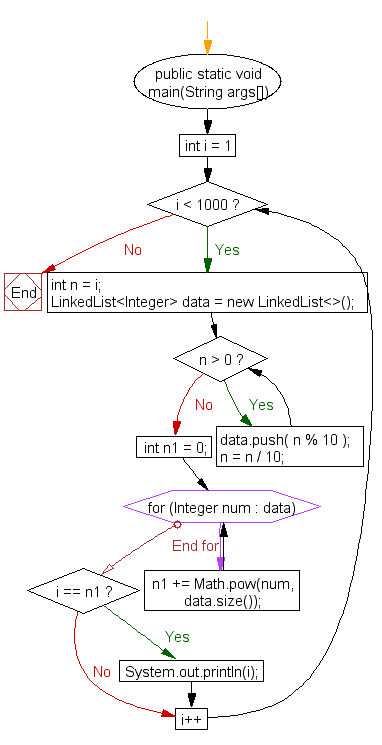﻿ Java: Find all the narcissistic numbers between 1 and 1000# Java Exercises: Find all the narcissistic numbers between 1 and 1000

## Java Numbers: Exercise-23 with Solution

Write a Java program to find all the narcissistic numbers between 1 and 1000.

In number theory, a narcissistic number is a number that is the sum of its own digits each raised to the power of the number of digits.
For example:
153 = 13 + 53 + 33

Pictorial Presentation:Sample Solution:

Java Code:

``````import java.util.LinkedList;
public class Example23  {

public static void main(String args[])
{
for (int i = 1; i < 1000; i++) {
int n = i;
while (n > 0) {
data.push( n % 10 );
n = n / 10;
}
int n1 = 0;
for(Integer num : data) {
n1 += Math.pow(num, data.size());
}
if(i == n1) {
System.out.println(i);
}
}
}
}
```
```

Sample Output:

```1
2
3
4
5
6
7
8
9
153
370
371
407
```

Flowchart:Java Code Editor:

What is the difficulty level of this exercise?

Test your Programming skills with w3resource's quiz.

﻿

## Java: Tips of the Day

How to convert a char array back to a string?

```char[] a = {'h', 'e', 'l', 'l', 'o', ' ', 'w', 'o', 'r', 'l', 'd'};
String b = new String(a);
```

Note however, that this is a very unusual situation: Because String is handled specially in Java, even "foo" is actually a String. So the need for splitting a String into individual chars and join them back is not required in normal code.

Ref: https://bit.ly/39x7Trn